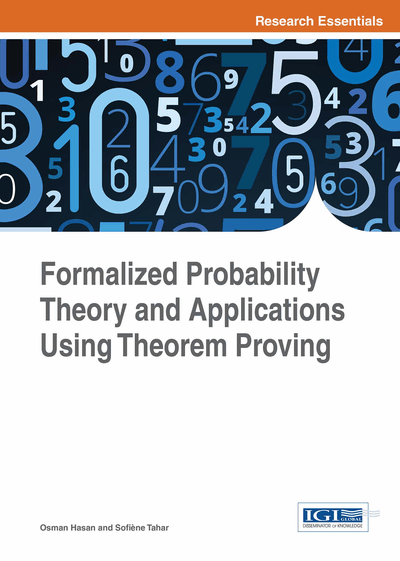# Classified Discrete-Time Markov Chains

DOI: 10.4018/978-1-4666-8315-0.ch007
Available
\$37.50
No Current Special Offers

## Abstract

The main focus of this chapter is on the formalization of classified DTMCs. The chapter begins by presenting the formalization of some foundational notions of classified states, which are categorized based on reachability, periodicity, or absorbing features. Then, these results along with the formal definition of a DTMC, presented in the previous chapter, are used to formalize classified Markov chains, such as aperiodic and irreducible DTMCs. Based on these concepts, some long-term properties are verified for the purpose of formally checking the correctness of the functions of Markovian systems or analyzing the performance of Markov chain models.
Chapter Preview
Top

## 7.1 Formalization Of Classified States

The foremost concept of states classification is the first passage time τj, which is sometimes referred to as the first hitting time. It is defined as the minimum time required to reach a state j from the initial state i:

τj = min { t > 0: Xt = j }(7.1)

The first passage time can be defined in HOL4 as:

• Definition 7.1

`Ⱶ ∀ X x j. FPT X x j = MINSET { t | 0 < t ∧ (X t x = j) }`

where X is a random process and x is a sample in the probability space associated with the random variable Xt. Note that the first passage time is also a random variable.

The conditional distribution of τj defined as the probability of the events starting from state i and visiting state j at time n is expressed as fij(n) = Pr { τj = n | X0 = i }.

This definition can be formalized in HOL4 as (Liu, 2013):

• Definition 7.2

```Ⱶ ∀ X p i j n.
f X p i j n = prob p ({ x | FPT X x j = n } | { x | X 0 x = i }) ```

Another important notion, denoted as fij, is the probability of the events starting from state i and visiting state j at all times n, is expressed as fij =fij(n) . It can be expressed in HOL4 as (λ n. f X p i j n) sums fij. Thus fjj provides the probability of events starting from state j and eventually returning back to j. If fjj = 1, then the mean return time of state j is defined as μj=n fjj(n). The existence of this infinite summation can be specified as summmable (λ n. n ∗ f X p j j n) in HOL.

A state j in a DTMC { Xt } t ≥ 0 is called transient if fjj < 1, and persistent if fjj = 1. If the mean return time μj of a persistent state j is finite, then j is said to be persistent non null state (or positive persistent state). Similarly, if μj is infinite, then j is termed as persistent null state.

The greatest common divisor (gcd) of a set is a frequently used mathematical concept in defining classified states. We formalize the gcd of a set as follows:

• Definition 7.3

`Ⱶ ∀A. GCD SET A = MAXSET { r | ∀x. x ∈A ⇒ divides r x }`

where MAXSET is a function in the set theory of HOL4 such that MAXSET s defines the maximum element in the set s. A period of a state j is any n such that pjj(n)is greater than 0 and we write dj = gcd { n: pjj(n) > 0 }as the gcd of the set of all periods.

A state i is said to be accessible from a state j (written i → j), if there exists a nonzero n-step transition probability of the events from state i to j. Two states i and j are called communicating states (written i ↔ j) if they are mutually accessible. A state j is an absorbing state if the one-step transition probability pjj =1. The formalization of some other foundational notions of the classified states is given in Table 1.

## Complete Chapter List

Search this Book:
Reset